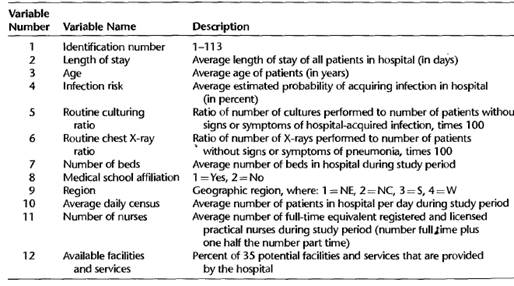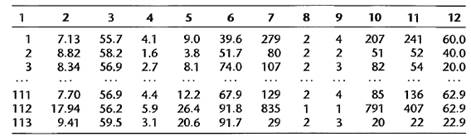# Refer to the SENIC data set in Appendix C.l and Project 1.46. For each geographic region, obtain… 1 answer below »

Refer to the SENIC data set in Appendix C.l and Project 1.46. For each geographic region, obtain the residuals and prepare a residual plot against X and a normal probability plot. Do the four regions appear to have similar error variances? What other conclusions do you draw from your plots?

Appendix C. I

The primary objective of the Study on the Efficacy of Nosocomial Infection Control (SENIC Project) was to determine whether infection surveillance and control programs have reduced the rates of nosocomial (hospital-acquired) infection in United States hospitals. This data set consists of a random sample of 113 hospitals selected from the original 338 hospitals

surveyed. Each line of the data set has an identification number and provides information on 11 other variables for a single hospital. The data presented here are for the 1975-76 study period. The 12 variables are:Project 1.46

Refer to the SENIC data set in Appendix C.1.

a. For each geographic region, regress average length of stay in hospital (Y) against infection risk (X). Assume that first-order regression model (1.1) is appropriate for each region. State the estimated regression functions.

b. Are the estimated regression functions similar for the four regions? Discuss.

c. Calculate MSE for each region. Is the variability around the fitted regression line approximately the same for the four regions? Discuss.